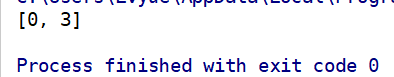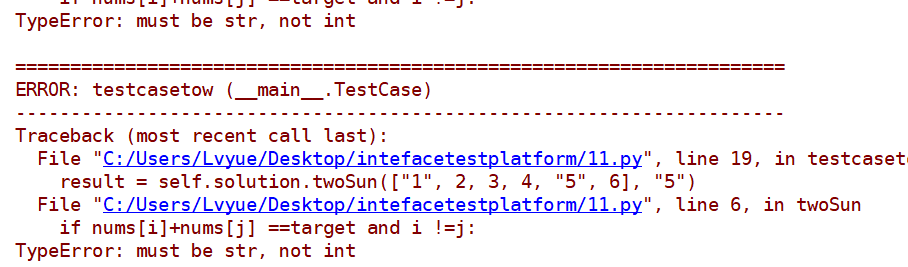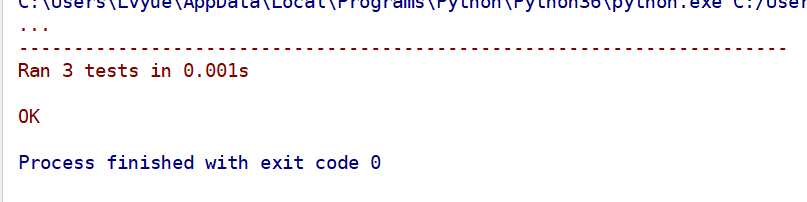# 刷题之给定一个整数数组 nums 和一个目标值 taget，请你在该数组中找出和为目标值的那 两个 整数

今天下午，看了一会github，想刷个题呢，就翻出来了刷点题提高自己的实际中的解决问题的能力，在面试的过程中，我们发现，其实很多时候，面试官 给我们的题，其实也是有一定的随机性的，所以我们要多刷更多的题。去发现问题。

题目：

    给定一个整数数组 nums 和一个目标值 taget，请你在该数组中找出和为目标值的那 两个 整数，并返回他们的数组下标。

实际这里就是给你的一个列表的数字，给你一个预期，让你返回

1.直接用到两个for循环，去遍历这个list，

2.一个for循环从第一个元素，一个for循环从减去这个元素的list里面去遍历

3.然后去判断这个两个的元素相加的和是否等于预期的taget,如果等于，直接返回元素的下标。

4. 这里可能返回多个，我们默认选择第一组。

class Solution():    def twoSun(self,nums,target):        for i in range(len(nums)):            for j in range(len(nums)):                if nums[i]+nums[j] ==target and i !=j:                    return [i,j]

if __name__=="__main__":    solution=Solution()    print(solution.twoSun([1,2,3,4,5,6],5))class TestCase(unittest.TestCase):    def setup(self):        pass    def tearDown(self) -> None:        pass    def testcaseone(self):        self.solution = Solution()        result=self.solution.twoSun([1, 2, 3, 4, 5, 6], 5)        self.assertEqual(result,[0,3])    def testcasetow(self):        self.solution = Solution()        result = self.solution.twoSun(["1", 2, 3, 4, "5", 6], "5")        self.assertEqual(result, [0, 3])    def testcasethree(self):        self.solution = Solution()        result = self.solution.twoSun(["a", 2, 3, 4, "b", 6], "ab")        self.assertEqual(result, False)if __name__=="__main__"：

unittest.main(）class Solution():    def twoSun(self,nums:list,target:int):        for i in range(len(nums)):            for j in range(len(nums)-i):                try:                    if nums[i]+nums[j] ==target and i !=j:                        return [i,j]                except:                    return Falseposted @ 2019-10-28 09:46  北漂的雷子  阅读(1204)  评论(0编辑  收藏